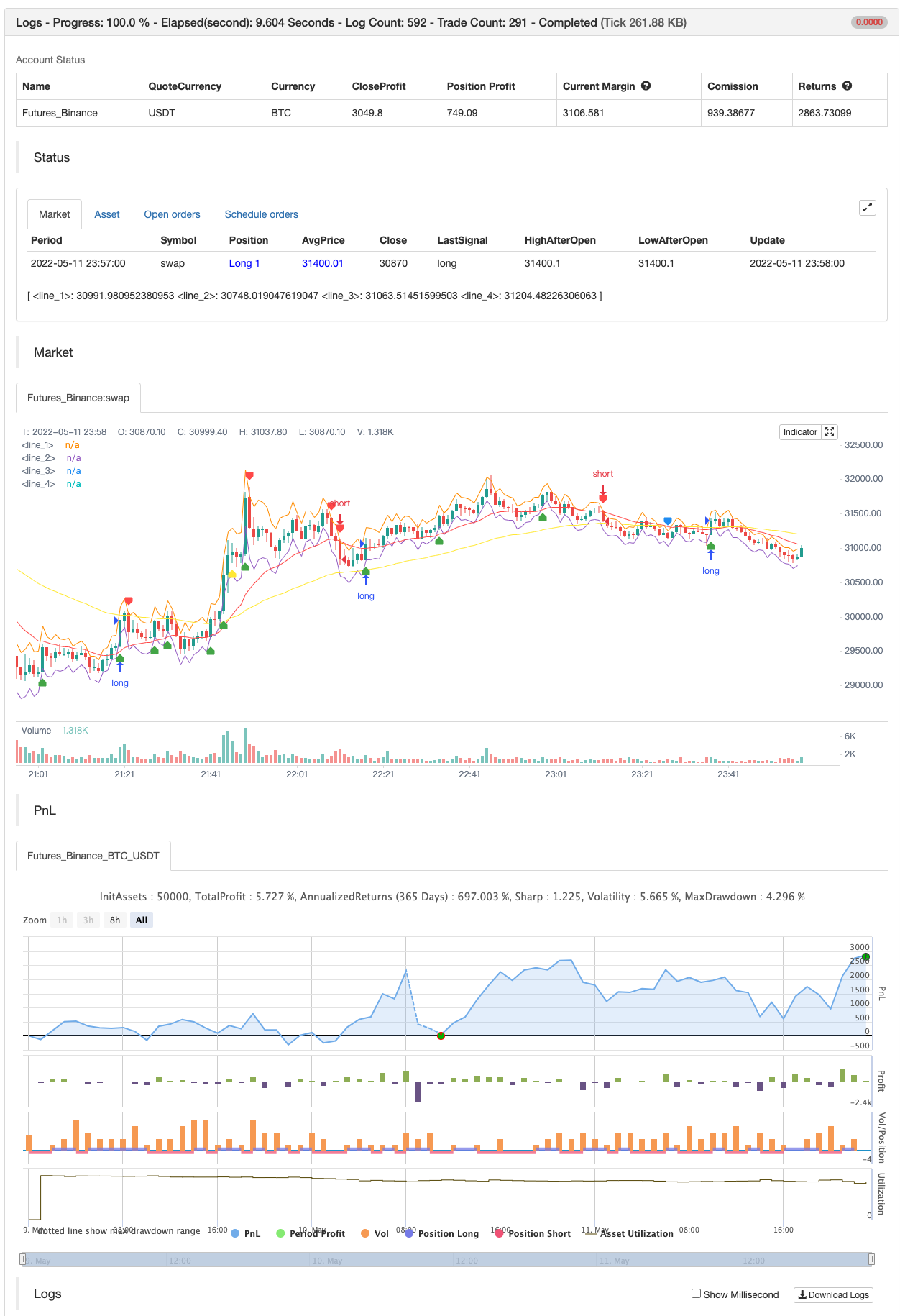# Super Scalper

Author: 张超, Date: 2022-05-17 14:23:28
Tags: RMA sma EMA WMA ATR

This strategy is based on RSI and ATR Bands which works better in 5 and 15 Mins time frame. Perform enough back testing with 1:2R before using in real time.

I have also added Golden Cross Over of 65 and 21 EMA to have confirmation on trend.

backtest```/*backtest
start: 2022-05-09 00:00:00
end: 2022-05-11 23:59:00
period: 1m
basePeriod: 1m
exchanges: [{"eid":"Futures_Binance","currency":"BTC_USDT"}]
*/

//@version=4
strategy("Super Scalper - 5 Min 15 Min", overlay=true)
source = close
atrlen = input(14, "ATR Period")
mult = input(1, "ATR Multi", step=0.1)
smoothing = input(title="ATR Smoothing", defval="WMA", options=["RMA", "SMA", "EMA", "WMA"])
ma_function(source, atrlen) =>
if smoothing == "RMA"
rma(source, atrlen)
else
if smoothing == "SMA"
sma(source, atrlen)
else
if smoothing == "EMA"
ema(source, atrlen)
else
wma(source, atrlen)
atr_slen = ma_function(tr(true), atrlen)
upper_band = atr_slen * mult + close
lower_band = close - atr_slen * mult

// Create Indicator's
shortSMA = ema(close, 21)
longSMA = ema(close, 65)
rsi = rsi(close, 14)
atr = atr(14)

// Specify  conditions
longCondition = open < lower_band
shortCondition = open > upper_band
GoldenLong = crossover(shortSMA,longSMA)
Goldenshort = crossover(longSMA,shortSMA)

plotshape(shortCondition, title="Sell Label", text="Sell", location=location.abovebar, style=shape.labeldown, size=size.tiny, color=color.red, textcolor=color.white, transp=0)
plotshape(Goldenshort, title="Golden Sell Label", text="Golden Crossover Short", location=location.abovebar, style=shape.labeldown, size=size.tiny, color=color.blue, textcolor=color.white, transp=0)
plotshape(GoldenLong, title="Golden Buy Label", text="Golden Crossover Long", location=location.belowbar, style=shape.labelup, size=size.tiny, color=color.yellow, textcolor=color.white, transp=0)
// Execute trade if condition is True
if (longCondition)
stopLoss = low - atr * 2
takeProfit = high + atr * 5
strategy.entry("long", strategy.long, when = rsi > 50)

else if (shortCondition)
stopLoss = high + atr * 2
takeProfit = low - atr * 5
strategy.entry("short", strategy.short, when = rsi < 50)

// Plot ATR bands to chart

////ATR Up/Low Bands

plot(upper_band)
plot(lower_band)

// Plot Moving Averages
plot(shortSMA, color = color.red)
plot(longSMA, color = color.yellow)
```

Related

More